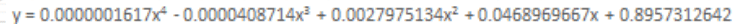# Transfer function from a fourth order polynomial?

• Secold

## Homework Statement

Excel data for an assignment I'm doing has spit out a curve from some experimental data as shown here:http://i.imgur.com/KcJyEEj.png

I'm wondering if there's a nice way to put this as a transfer function in the form of Y/X or something similar

## The Attempt at a Solution

Simply using algebra seemed to not work to be able to get y/x, unless I'm missing something obvious.
I figured maybe if I used laplace transforms I could get a situation where Y/X would appear. Not sure how to go about this as it's pretty different from the differential equations laplace is normally used on.

Any idea?

The polynomial is probably the only way to express it, though you could factorize it if you consider that would look neater.

Maybe a quartic is too unwieldy for you? I'd expect your real life process will be limited to a restricted range of x values, so you could try approximating your quartic over that useful range with a cubic or even a binomial. You might find you can get a good fit.

The usual "transfer function" involves both magnitude and phase, each smoothly changing with frequency. You have no phase term here, apart from maybe a couple of abrupt sign reversals as the graph crosses the x-axis.

## Homework Statement

Excel data for an assignment I'm doing has spit out a curve from some experimental data as shown here:http://i.imgur.com/KcJyEEj.png

I'm wondering if there's a nice way to put this as a transfer function in the form of Y/X or something similar
No, since the relationship is nonlinear. A transfer function needs to be linear, by definition. You can approximate nonlinear relationships by describing functions, but this is not a true transfer function.

Some people might take your expression and call it a transfer function. Not common usage but OK IMO.

https://en.wikipedia.org/wiki/Transfer_function